# Catch up on the latest AI articles# Time Series Forecasting Using An Energy-based Generative Model That Has Recently Attracted Much Attention

3 main points
✔️ Propose a multivariate time series forecasting framework ScoreGrad
✔️ Uses an energy-based generative model and score matching
✔️ Verify SOTA performance using real-world datasets

The images used in this article are from the paper or created based on it.

## first of all

A wide range of sensors is used to record the state of increasingly complex systems. They are treated as multivariate data with correlations. With the development of deep learning, multivariate time series forecasting has made significant progress.

On the other hand, there are some limitations. These include the inability to model probabilistic information in time series, and the inability to model long-term time dependence.

The limitations of the EBM (Energy-Based generative Model) based TimeGrad have been weakened, but still have the following limitations: 1) the DDPM (Denoised Diffusion Probabilistic Model) used in TimeGrad is sensitive to the size of the noise injected into the original distribution. distribution; 2) the number of steps used for noise injection must be carefully designed; 3) the sampling method of the generative process can be further extended with DDPM, and 4) the number of steps used for noise injection must be carefully designed.

To solve these problems, we propose ScoreGrad, a general framework for multivariate time series forecasting based on continuous energy-based generative models.

1) ScoreGrad is the first to apply a continuous energy-based generative model to multivariate time series forecasting.

2) The learning process of each step consists of extraction of feature values of the time series and conditional SDE (Stochastic Differential Equation ) by score matching module. The prediction is done by solving the inverse time SDE.

3) ScoreGrad was applied to the prediction of six real-world datasets to check the SOTA performance.

## related research

### multivariate time series forecasting

Following statistical methods such as ARIMA, deep learning methods have been studied and DeepAR, MQRNN, etc. have been proposed. In addition, there are RNNs combined with attention, residual, and dilatation coupling. Recently, probabilistic models, which explicitly model data distributions with normalized flows or generative models with GANs, have shown better performance than deterministic models. However, the functional form of these methods has limitations, and some are sensitive to hyperparameters.

### Energy-based generation model

The Energy Based Model (EBM), also promoted by Professor Yann LeCun, is an unnormalized stochastic model. The output is a scalar: a small value if the two inputs are close together, and a large value if they are far apart. The following material is from a deep learning lecture at New York University.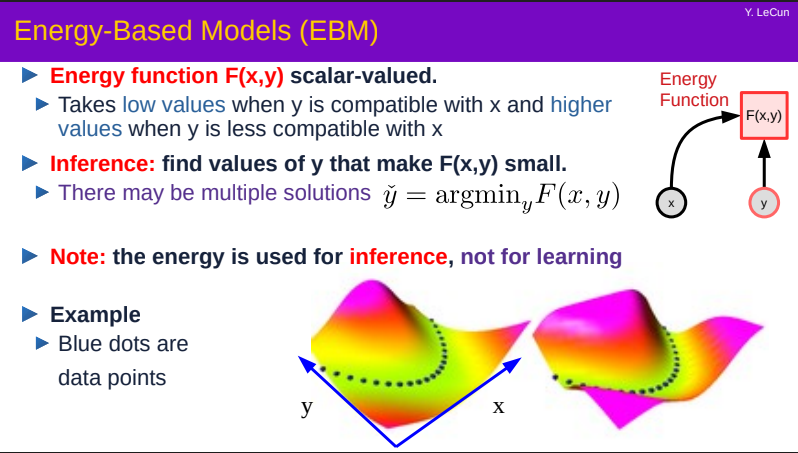EBMs are much less restrictive in their functional form and have a wide range of applications in various domains such as natural language processing and density estimation. However, the unknown normalization constants of EBMs make them difficult to learn. The following is the current learning method.

1) MCMC maximum likelihood estimation: Instead of calculating the likelihood directly, MCMC sampling methods such as Hamiltonian Monte Carlo are used to estimate the log-likelihood gradient.

Fisher divergence to Minimize the deviation of the gradient of the log-likelihood between the data distribution and the estimated distribution.

3) Noise contrast estimation: The concept is that an EBM can be learned by contrasting it with known densities.

In this paper, we focus on the second EDM with score matching. Inspired by , a continuous SDE-based energy-based model for image generation models, we apply it to multivariate time series forecasting.

## score-based generative model

### Score-matching model

Instead of using maximum likelihood estimation, score matching tries to minimize the distance of the log density function derivative between the data and the model distribution. Although the density function of the data distribution cannot be known, the objective can be simplified by a trick of integration by parts to equation 1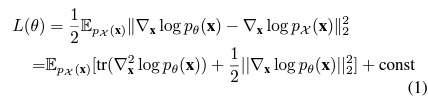The $\nabla_x logp_\theta (x)$ is called the score function.

### discrete score matching model

Recently, two classes of energy-based generative models that use various levels of noise to estimate the scoring network have achieved good performance in image generation tasks, and are structured as in Fig. 1, which describes the processes of forwarding and backpropagation.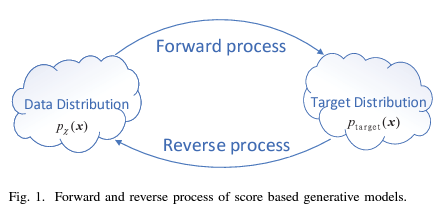Score matching in Langevin dynamics

SMLD (Score matching with Langevin dynamics) is a method to improve the score-based generative model by perturbing the data with various levels of noise, and NCSN (Noise Conditioned Score Network) to estimate scores for all noise levels.

The definition of the oscillating kernel is equation (2). The noise sequence is in ascending order ${\sigma_1, \sigma_2, \cdots , \sigma_N}$.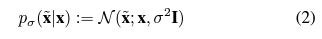For generation, Langevin MCMC is used for iterative sampling. With the number of iterative steps as M, the sampling process of $p_\sigma _i (x)$ can be formulated as follows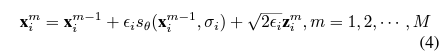Denoising diffusion stochastic model

The noise sequence is 0<$\beta _i$<1, i=1,2,$\cdots$, N and the discrete Markov chain is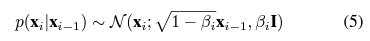The backpropagation process is by inverse Markov chain, which is This method is called propagation sampling. [10 ]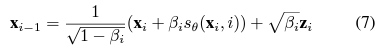### Score matching in SDE

In , it is shown that the above two noise-containing processes can be modeled in stochastic numerical form. Without loss of generality, the SDE can be considered as follows: w denotes the standard Wiener process; f is the drift coefficient; g is a scalar function called the diffusion coefficient.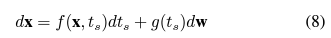The inverse process of (8) also satisfies the SDE and is of the form At the same time, the above two can be treated as discrete forms of continuous-time SDE.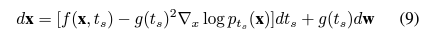Table 1 summarizes the results.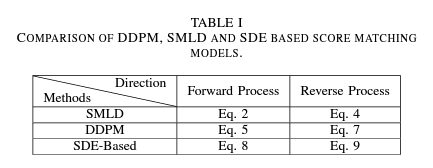The following three SDEs are used in ScoreGrad.

VE SDE (Variance Exploding) is so-called because the variables explode when t goes to infinity.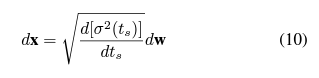In VP SDE (Variance Preserving), N goes to infinity in equation (5), which leads to equation (11). The upper bound of $\Sigma (t)$ is always $\Sigma (0)$.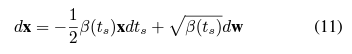In sub-VP SDE, the upper bound of a variable always corresponds to the VP SDE.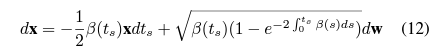## technique

### Symbols and Problem Formulation

Let $\Kai$= {$x_1^0, x_2 ^0, \cdots , x_T ^0$} be a multivariate time series in D dimensions. The probabilistic forecasting task can be translated into a forecast of $q_\Kai$.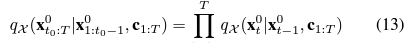### model architecture

The general framework of ScoreGrad is shown in Fig. 2. It consists of two parts: the time series feature value extraction module in the left half and the conditional stochastic differential equation (SDE) based score matching module in the dotted line in the right half.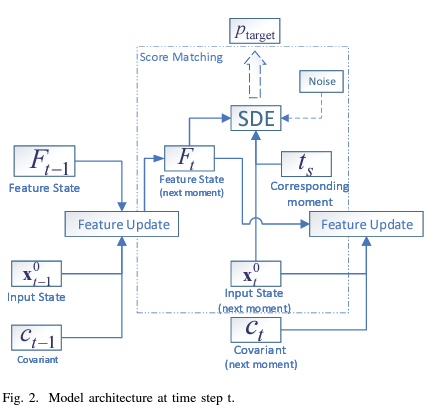Time Series Feature Value Extraction Module Time series feature value extraction module

The feature value Ft is sequentially updated by the update function R based on the past data.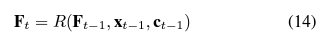It is a general framework and can use many sequence model methods: RNN, GRU, TCN, etc. The iterative prediction strategy in (13) can be transformed into the following conditional prediction problem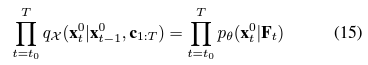In this paper, a recurrent neural network is used as a default.

Conditional SDE-based Score Matching Module

As shown in Fig.3, Ft is used as a conditioner for the SDE-based score matching model at each time point. The forward propagation follows equation (8) and the backpropagation follows the equation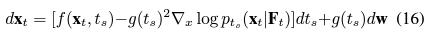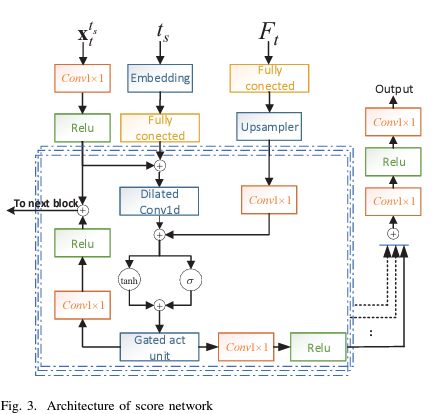### conditional score network

Following WaveNet and DiffWave, the conditional score network has eight residual blocks; Fig. 3 shows a single block. The embedding is not positional, but random Fourier feature value embedding.

### learning

Each module is trained with the following loss function using the loss functions of SMLD and DDPM described earlier.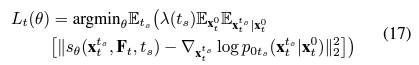### prediction

The prediction process is an iterative sampling from an inverse continuous time SDE. See Fig. 4 for details at each time step. As a sampler, we follow  and use a PC (Predictor-corrector) sampler.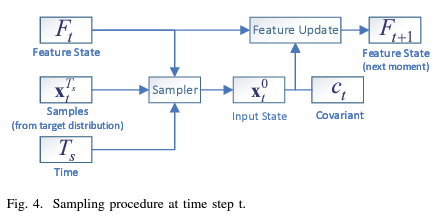## Experiment

### Data sets, evaluation indicators

We use the six datasets shown in Table II for the evaluation. We use the Continuous Ranked Probability Score (CRPS) for each time series dimension and CRPSsum for the sum of the time series dimensions, which is used to measure the compatibility of the cumulative distribution function (CDF).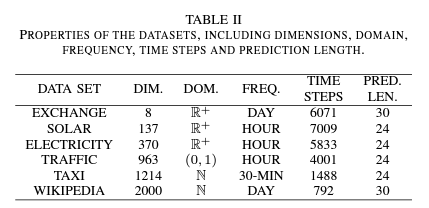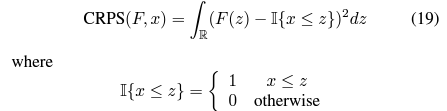### comparative method

There are eight objects for comparison. Without going into details, these methods are based on autoregressive, LSTM, Kalman filter, and energy-based models.

### Result

We evaluated three SDEs and eight comparators in the ScoreGrad framework and the results are shown in Table III: the mean and standard deviation of CRPS. The deep learning method performs better than the statistical method. The dimension of the latent variable has a noticeable impact on the performance; TimeGrad replaces the normalized flow with DDPM, which gives better results than all but Exchange.

The three ScoreGrad methods have the best results outside of the Exchange dataset; VP SDE is better than VE SDE; VE SDE is better on the Traffic dataset; VP SDE is better than VE SDE, and VE SDE is better on the Exchange dataset.